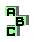CENTROID    (1/10)

 r3 first Brocard triangle, similar triangles r8 pedal triangle, second Brocard triangle, similar triangles r11 first Brocard triangle, second Brocard triangle, perspective r19 point X(110), Brocard circle, inverse points r20 first Brocard triangle, Euler line, Tarry point r24 Steiner point, symmedian point, tripole r28 Fermat points, Euler line r35 isodynamic points, Fermat points, Euler line r41 Vecten points, isodynamic points, Fermat points, symmedian point, orthocenter, circumcenter, Brocard line, De Longchamps point, point X(376), autopolar triangle d Parry circle r44 perspector, cevian quotient , circumconic r47 cevian product, symmedian point, Kiepert hyperbola r65 incenter, Nagel point, Spieker point r72 incenter, point X(165), Nagel point, De Longchamps point r68 Nagel point, incircle, Spieker circle r55 point X(115), point X(125), ninecircle, pedal circle d anticomplement d complement d Kiepert hyperbola d Lemoine ellipse d Steiner circles d Steiner inellipse d Steiner circumellipse d McCay circles1 2 3 4 5 6 7 8 9 10